Auto ByteScience AI# 理解XGBoost机器学习模型的决策过程

``````import pandas as pd
from xgboost import XGBClassifier
from sklearn.model_selection import train_test_split
from sklearn.metrics import accuracy_score
import operator
import matplotlib.pyplot as plt
import seaborn as sns
import lime.lime_tabular
from sklearn.pipeline import Pipeline
from sklearn.preprocessing import Imputer
import numpy as np
from sklearn.grid_search import GridSearchCV
%matplotlib inline```
```

• 清除名字和乘客 ID
• 把分类变量转化为虚拟变量
• 用中位数填充和去除数据

``````data = pd.read_csv("./data/titantic/train.csv")
y = data.Survived
X = data.drop(["Survived", "Name", "PassengerId"], 1)
X = pd.get_dummies(X)```
```

````X_train, X_test, y_train, y_test = train_test_split(X, y, test_size=0.33, random_state=42)`
```

``````pipeline = Pipeline([('imputer', Imputer(strategy='median')), ('model', XGBClassifier())])
parameters = dict(model__max_depth=[3, 5, 7],
model__learning_rate=[.01, .1],
model__n_estimators=[100, 500])
cv = GridSearchCV(pipeline, param_grid=parameters)
cv.fit(X_train, y_train)```
```

``````test_predictions = cv.predict(X_test)
print("Test Accuracy: {}".format(accuracy_score(y_test, test_predictions)))
Test Accuracy: 0.8101694915254237```
```

``````fi = list(zip(X.columns, cv.best_estimator_.named_steps['model'].feature_importances_))
fi.sort(key = operator.itemgetter(1), reverse=True)
top_10 = fi[:10]
x = [x for x in top_10]
y = [x for x in top_10]```
```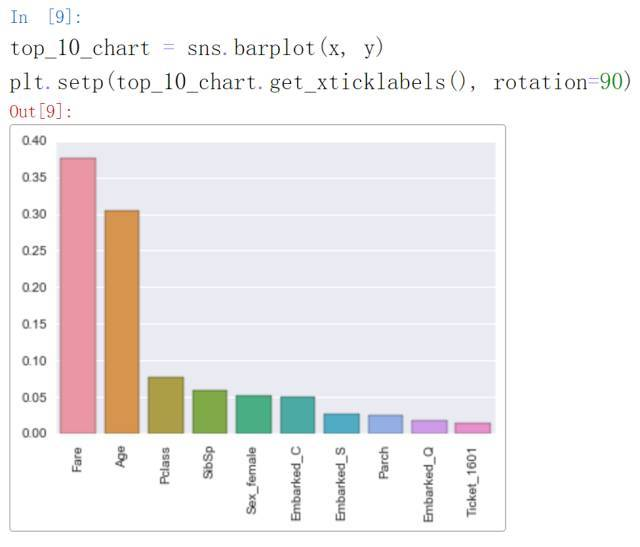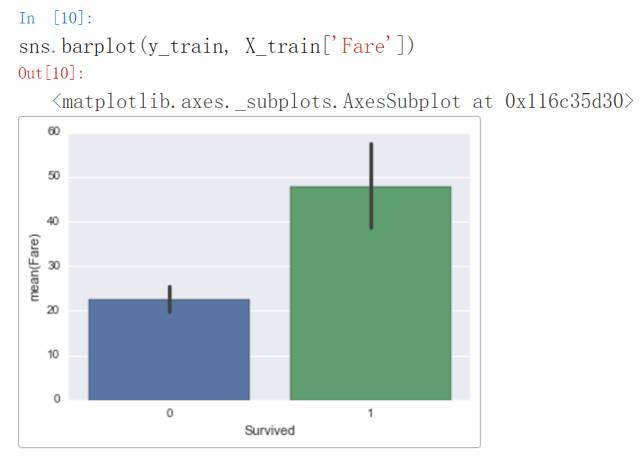``````X_train_imputed = cv.best_estimator_.named_steps['imputer'].transform(X_train)
explainer = lime.lime_tabular.LimeTabularExplainer(X_train_imputed,
feature_names=X_train.columns.tolist(),
class_names=["Not Survived", "Survived"],
discretize_continuous=True)```
```

``````model = cv.best_estimator_.named_steps['model']
def xgb_prediction(X_array_in):
if len(X_array_in.shape) < 2:
X_array_in = np.expand_dims(X_array_in, 0)
return model.predict_proba(X_array_in)```
```

``````X_test_imputed = cv.best_estimator_.named_steps['imputer'].transform(X_test)
exp = explainer.explain_instance(X_test_imputed, xgb_prediction, num_features=5, top_labels=1)
exp.show_in_notebook(show_table=True, show_all=False)```
```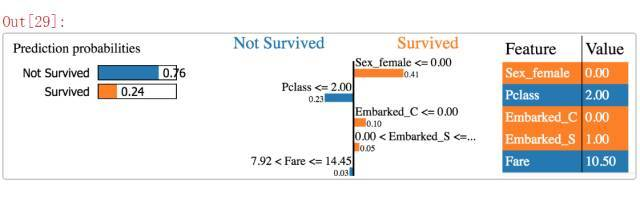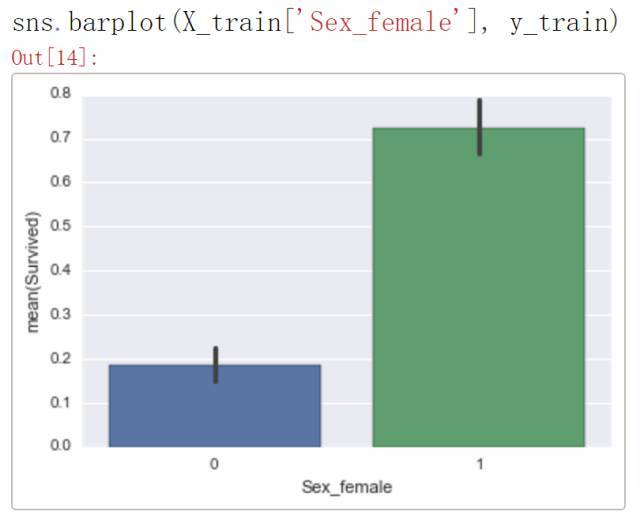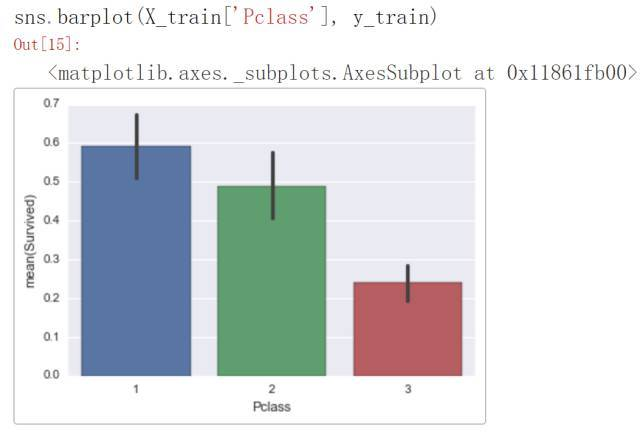``````y_test.values
>>>1```
```